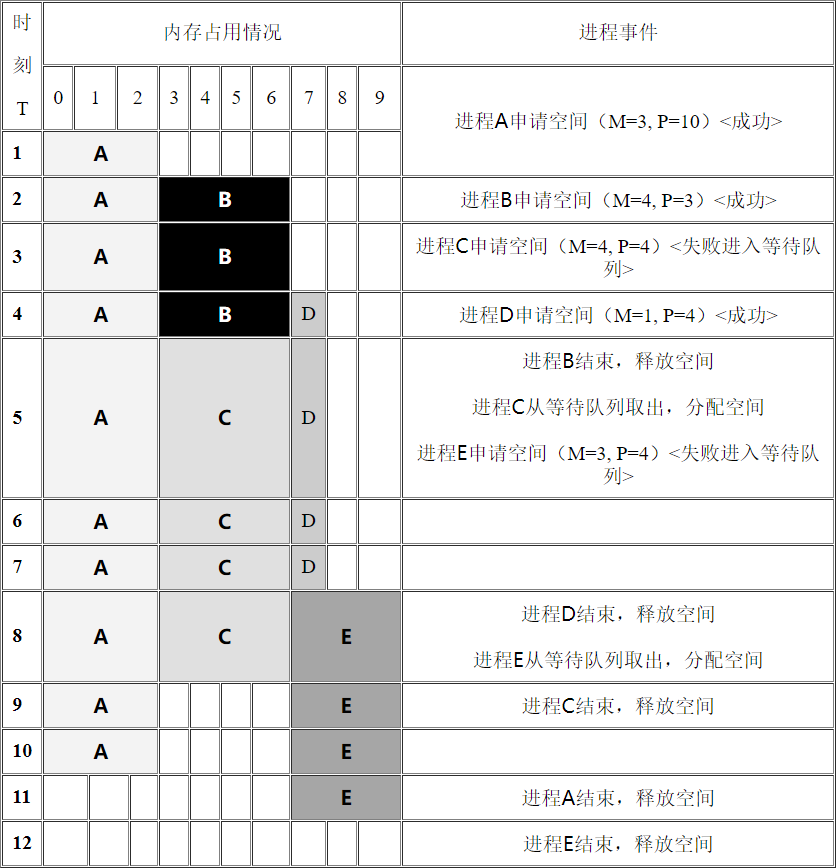155. 内存分配

1、 内存以内存单元为基本单位，每个内存单元用一个固定的整数作为标识，称为地址。地址从 $0$ 开始连续排列，地址相邻的内存单元被认为是逻辑上连续的。我们把从地址 $i$ 开始的 $s$ 个连续的内存单元称为首地址为 $i$ 长度为 $s$ 的地址片。

2、 运行过程中有若干进程需要占用内存，对于每个进程有一个申请时刻 $T$，需要内存单元数 $M$ 及运行时间 $P$。在运行时间 $P$ 内（即 $T$ 时刻开始，$T+P$ 时刻结束），这 $M$ 个被占用的内存单元不能再被其他进程使用。

3、假设在T时刻有一个进程申请 $M$ 个单元，且运行时间为 $P$，则：

1. 若 $T$ 时刻内存中存在长度为 $M$ 的空闲地址片，则系统将这 $M$ 个空闲单元分配给该进程。若存在多个长度为 $M$ 个空闲地址片，则系统将首地址最小的那个空闲地址片分配给该进程。

2. 如果 $T$ 时刻不存在长度为 $M$ 的空闲地址片，则该进程被放入一个等待队列。对于处于等待队列队头的进程，只要在任一时刻，存在长度为 $M$ 的空闲地址片，系统马上将该进程取出队列，并为它分配内存单元。注意，在进行内存分配处理过程中，处于等待队列队头的进程的处理优先级最高，队列中的其它进程不能先于队头进程被处理。

#### 输入样例：

10
1 3 10
2 4 3
3 4 4
4 1 4
5 3 4
0 0 0


#### 输出样例：

12
2


#### 提示